# R-style Visualizations in PythonVisualizations are a great way to quickly understand a new dataset. They make it easier to identify correlations between the various columns, as well as identify informative patterns in the data.

Visualizations are a great way to quickly understand a new dataset. They make it easier to identify correlations between the various columns, as well as identify informative patterns in the data. There are several visualization libraries available for Python users such as matplotlib, seaborn, plotly, and graphiz. Since both R and Python are commonly used in data science and analytics, you may find yourself going between both languages. Maybe your organization is converting projects from R to Python, or you are an R user that has joined a team that works exclusively in Python. Or perhaps you have come across something done in R and simply wondered if it could be implemented in Python.

Ggplot is a commonly used library in R for data visualization. The Python equivalent is plotnine. This article will explore using plotnine for basic visualizations and will conclude with pros and cons of implementation. This exploration also assumes a basic knowledge of Python and its frequently used libraries such as pandas for data manipulation.

## Understand and load the data

This exploration is based on the 2014 Uber dataset hosted on Kaggle. The four columns in the data are:

• `Date/Time` : The date and time of the Uber pickup
• `Lat` : The latitude of the Uber pickup
• `Lon` : The longitude of the Uber pickup
• `Base` : The TLC (Taxi & Limousine Commission) base company code affiliated with the Uber pickup

To demonstrate plotnine, we will focus on the Data/Time column. First, load the data into pandas data frames and concatenate them into one data frame. We will also need to convert the Date/Time column to datetime data type so that we can extract useful information from it.

``````apr_data = pd.read_csv('data/uber-raw-data-apr14.csv')
data = pd.concat([apr_data, may_data, jun_data, jul_data, aug_data, sep_data])
data['Date/Time'] = pd.to_datetime(data['Date/Time'], format='%m/%d/%Y %H:%M:%S')
``````

The next step is to create several time-dependent columns and a trip count column to make it easier to group the data by various time units.

create_columns.py

``````data['Day'] = data['Date/Time'].dt.day
data['Month'] = data['Date/Time'].dt.month
data['Year'] = data['Date/Time'].dt.year
data['Day_of_Week'] = data['Date/Time'].dt.dayofweek
data['Day_Name'] = data['Day_of_Week'].map({0: 'Mon', 1: 'Tue', 2: 'Wed', 3: 'Thur', 4: 'Fri', 5: 'Sat', 6: 'Sun'})
data['Month_Name'] = data['Month'].map({4: 'Apr', 5: 'May', 6: 'Jun', 7: 'Jul', 8: 'Aug', 9: 'Sep'})
data['hour'] = data['Date/Time'].dt.hour
data['minute'] = data['Date/Time'].dt.minute
data['second'] = data['Date/Time'].dt.second
data['trip_count'] = 1

``````

## Create visualizations

Now it’s time to create some graphs. For all the plots created, some manipulation of the original data frame with Pandas groupby method was required. It is cleaner to provide ggplot with a smaller data frame that only contains the necessary columns.

first_plot.py

``````# Group data
hour_data = data.groupby(by='hour').sum().reset_index()
hour_data = hour_data[['hour', 'trip_count']]

# Create plot
ggplot(hour_data, aes('hour', 'trip_count')) + \
geom_bar(data=hour_data, stat='identity', fill='#003f5c', color='#bc5090') + \
labels.ggtitle('Trips By Hour (Apr-Sep 2014)') + labels.xlab('Hour of Day') + labels.ylab('Total Trips') + \
scales.scale_x_continuous(breaks=range(0, 24)) + \
scales.scale_y_continuous(breaks=range(0, 400000, 50000))
``````

The output of the code block above is the graph below, which shows trips by hour of the day for the 6-month period.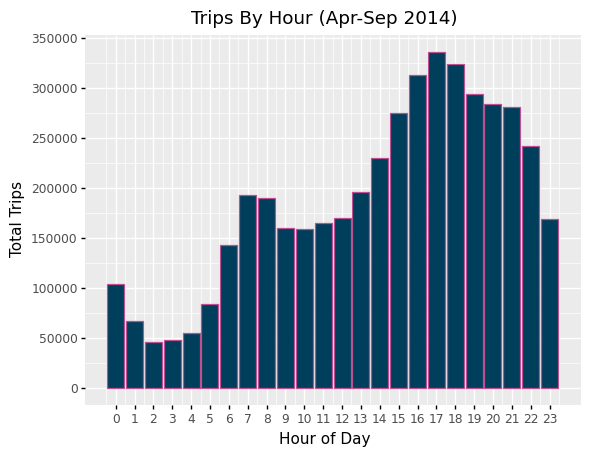The graph clearly shows when more rides are completed, beginning at the evening rush hours. The cool thing about easily-interpreted visualizations is that they lead to more questions, especially in the data exploration phase. The first chart may make you curious about whether this information is dependent on the month, day of the month, day of the week, etc. so let’s take a look at another configuration.

second_plot.py

``````month_hour = data.groupby(by=['Month', 'Month_Name', 'hour']).sum().reset_index()
month_hour = month_hour[['Month', 'Month_Name', 'hour', 'trip_count']]
ggplot(month_hour, aes('hour', 'trip_count', fill='Month')) + \
geom_bar(stat='identity') + \
labels.ggtitle('Trips By Hour and Month (Apr-Sep 2014)') + labels.xlab('Hour of Day') + labels.ylab('Total Trips') + \
scales.scale_x_continuous(breaks=range(0, 24)) + \
scales.scale_y_continuous(breaks=range(0, 400000, 50000))
``````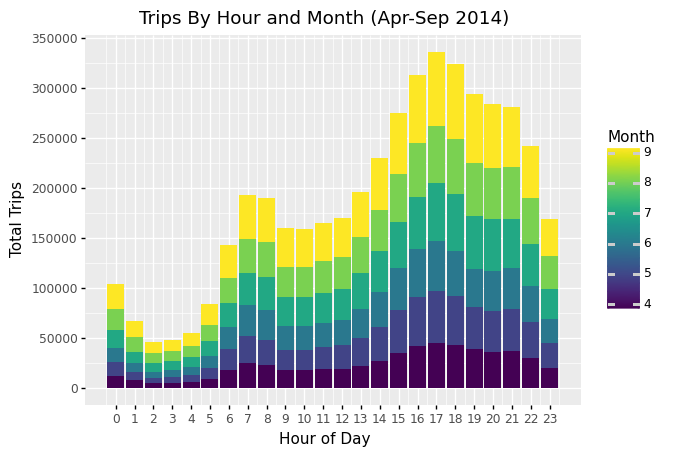September seems to have more trips, especially during evening hours but it is a little difficult to tell in this format. A heatmap would make it easier to see relationships.

heatmap.py

``````ggplot(month_hour, aes('hour', 'Month', fill='trip_count')) + \
geom_tile(color='white') + \
labels.ggtitle('Heatmap by Month and Hour of Day') + labels.ylab('Month') + labels.xlab('Hour of Day') + \
scales.scale_x_continuous(breaks=range(0, 24, 2)) + \
scales.scale_y_continuous(breaks=range(4, 10), labels=['Apr', 'May', 'Jun', 'Jul', 'Aug', 'Sep'])
``````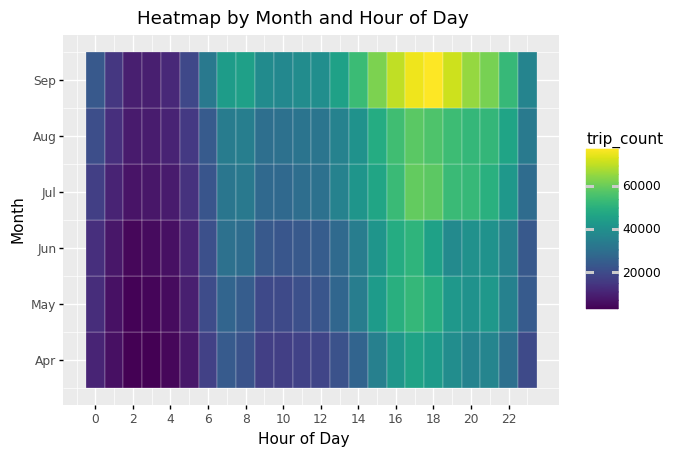The heatmap confirms the higher number of rides during early evening hours in September, which should prompt further investigation that is outside the scope of this dataset.

With a few minor changes, the code snippets provided above can be manipulated to investigate any other configuration that one is curious about.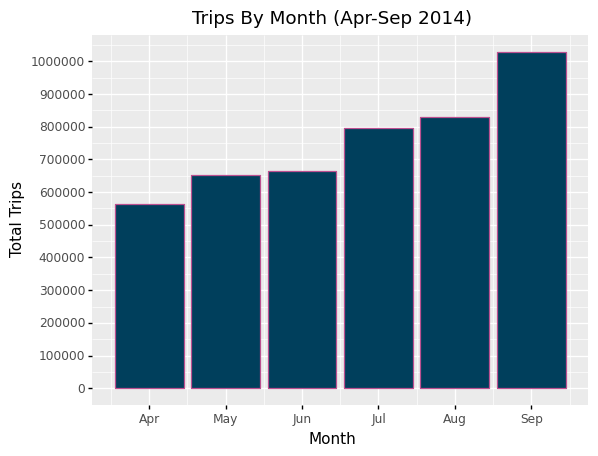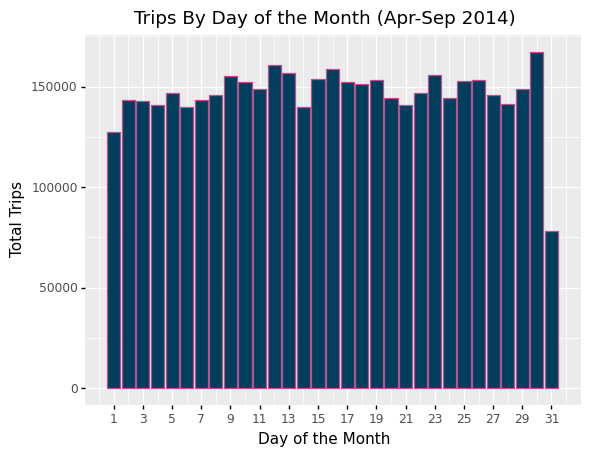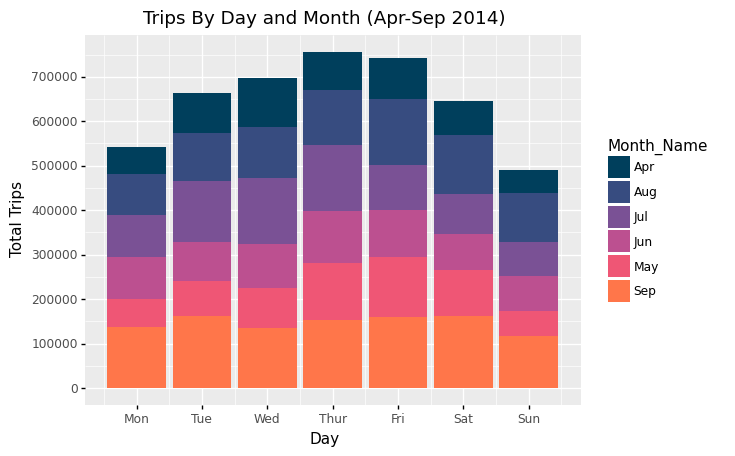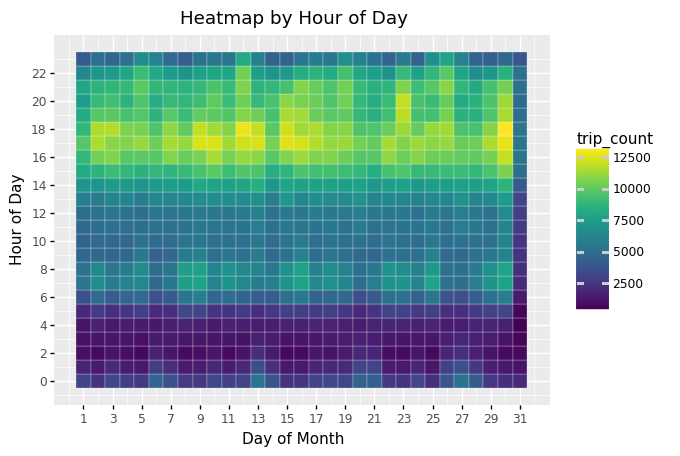## Conclusion

While these visualizations could be created with more commonly used Python libraries, here are the pros and cons of using plotnine when looking for a ggplot-like experience:

Pros

1. The plotnine documentation is fairly easy to understand and implement.
2. If you are coming to Python from R, or converting R to Python, it is likely easier to use plotnine than to try to do the same thing in a different library.
3. If you need to present your plots or share findings with collaborators, plotnine offers a save method.

Cons

1. Some of the functionality available in ggplot is not available in plotnine — for example creating maps using theme_map.
2. For common functionality, implementation does not always directly translate from R to Python so be prepared to spend time digging through the plotnine documentation (but this could be considered a pro from a learning perspective).
3. R offers a data frame manipulation library (dplyr) that makes it easy to chain actions that you would like to perform on a data frame prior to visualization. The Python equivalent, dfply, is less intuitive. For the few steps required in this project though, using pandas methods worked just fine.

The full implementation of this project can be found here.